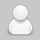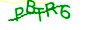×

# C++当函数返回值为类类型时 作为左值的疑问

#####来源于网络发表于2020-09-29 00:38:50浏览19评论0

#include
using namespace std;

//定义类

class Person
{
private:
int m_Age;
public:

//定义默认构造函数

Person()

{

cout << "默认构造函数！" << endl;

}

```//定义有参构造函数
Person(int age)
{
m_Age = age;

cout << "有参构造函数！" << endl;
}
```

//定义拷贝构造函数

Person(const Person& p)

```{

m_Age = p.m_Age;

cout << "拷贝构造函数！" << endl;
}
~Person()
{
cout << "析构函数！" << endl;
}
```

};

//定义返回值为Person的函数

Person func()

{

```Person p(10);

return p;
```

}

int main()

{

```func()= 10;//调用func函数，并将它的返回值作为左值
system("pause");
return 0;
```

}

```首先，你的程序能正常运行，你可以debug运行，将func() = 10;设断点，然后F11逐语句执行。

#include <iostream>

using namespace std;

//定义类

class Person
{
private:
int m_Age;
public:

//定义默认构造函数

Person()

{
m_Age = 0;
cout << "默认构造函数！" << endl;
cout << this << endl;   // 输出该对象的指针
cout << "m_Age = " << m_Age << endl << endl;
}

//定义有参构造函数
Person(int age)
{

m_Age = age;
cout << "有参构造函数！" << endl;
cout << this << endl;   // 输出该对象的指针
cout << "m_Age = " << m_Age << endl<< endl;

}
//定义拷贝构造函数

Person(const Person& p)

{

m_Age = p.m_Age;
cout << "拷贝构造函数！" << endl;
cout << this << endl;   // 输出该对象的指针
cout << "m_Age = " << m_Age << endl<<endl;
}
~Person()
{
cout << this << endl;   // 输出该对象的指针
cout << "析构函数！" <<"   "<<m_Age<<endl;
}
};

//定义返回值为Person的函数

Person func()

{

Person p(10);

return p;
}

int main()
{
func() = 20;//调用func函数，并将它的返回值作为左值，为了区别p(10),令func() = 20;
system("pause");
}

00EFF898
m_Age = 20

00EFF85C
m_Age = 10

00EFF8A4
m_Age = 10

00EFF85C

00EFF8A4

00EFF898

->
Person p = func();
p = 10;

【版权说明：本篇文章基于互联网CC-BY-NC-SA分享协议；如需转载时请注明出处及相应链接！】### 评论一下